# 用Python给头像加上圣诞帽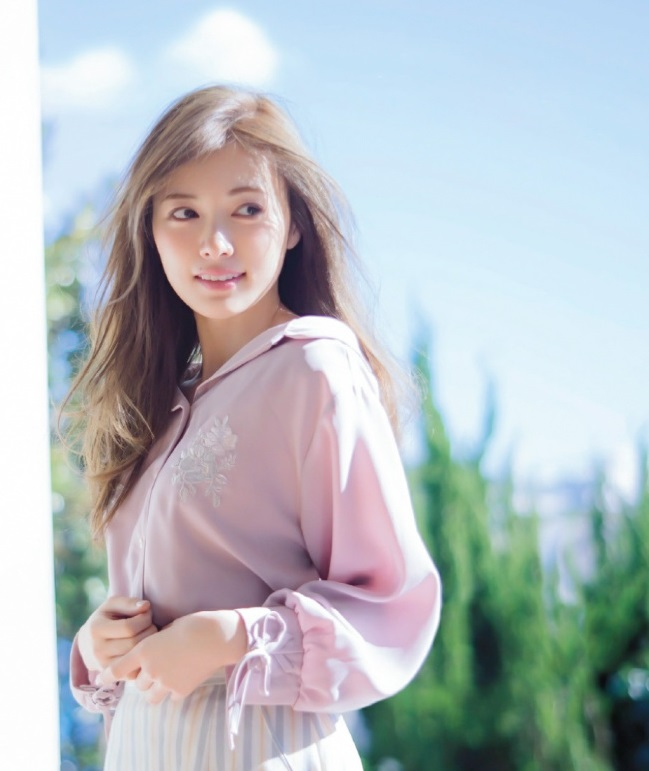### 用到的工具

• OpenCV（毕竟我们主要的内容就是OpenCV…）
• dlib（前一篇文章刚说过，dlib的人脸检测比OpenCV更好用，而且dlib有OpenCV没有的关键点检测。）

### 流程

#### 一、素材准备r,g,b,a = cv2.split(hat_img)
rgb_hat = cv2.merge((r,g,b))

cv2.imwrite("hat_alpha.jpg",a)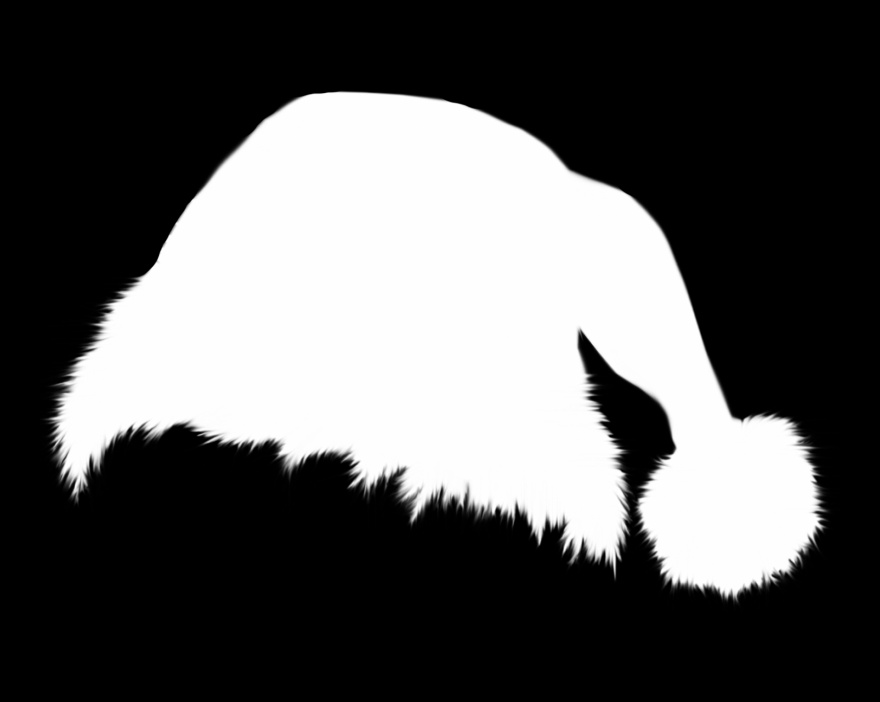#### 二、人脸检测与人脸关键点检测# dlib人脸关键点检测器
predictor_path = "shape_predictor_5_face_landmarks.dat"
predictor = dlib.shape_predictor(predictor_path)

# dlib正脸检测器
detector = dlib.get_frontal_face_detector()

# 正脸检测
dets = detector(img, 1)

# 如果检测到人脸
if len(dets)>0:
for d in dets:
x,y,w,h = d.left(),d.top(), d.right()-d.left(), d.bottom()-d.top()
# x,y,w,h = faceRect
cv2.rectangle(img,(x,y),(x+w,y+h),(255,0,0),2,8,0)

# 关键点检测，5个关键点
shape = predictor(img, d)
for point in shape.parts():
cv2.circle(img,(point.x,point.y),3,color=(0,255,0))

cv2.imshow("image",img)
cv2.waitKey()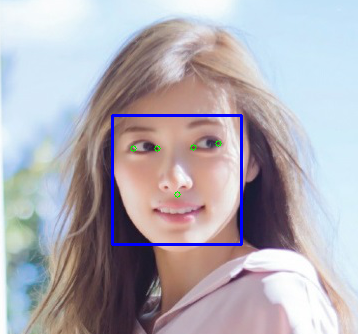### 三、调整帽子大小

            # 选取左右眼眼角的点
point1 = shape.part(0)
point2 = shape.part(2)

# 求两点中心
eyes_center = ((point1.x+point2.x)//2,(point1.y+point2.y)//2)

# cv2.circle(img,eyes_center,3,color=(0,255,0))
# cv2.imshow("image",img)
# cv2.waitKey()

#  根据人脸大小调整帽子大小
factor = 1.5
resized_hat_h = int(round(rgb_hat.shape*w/rgb_hat.shape*factor))
resized_hat_w = int(round(rgb_hat.shape*w/rgb_hat.shape*factor))

if resized_hat_h > y:
resized_hat_h = y-1

# 根据人脸大小调整帽子大小
resized_hat = cv2.resize(rgb_hat,(resized_hat_w,resized_hat_h))

#### 四、提取帽子和需要添加帽子的区域

            # 用alpha通道作为mask
mask_inv =  cv2.bitwise_not(mask)

            # 帽子相对与人脸框上线的偏移量
dh = 0
dw = 0
# 原图ROI
# bg_roi = img[y+dh-resized_hat_h:y+dh, x+dw:x+dw+resized_hat_w]
bg_roi = img[y+dh-resized_hat_h:y+dh,(eyes_center-resized_hat_w//3):(eyes_center+resized_hat_w//3*2)]

# 原图ROI中提取放帽子的区域
bg_roi = bg_roi.astype(float)

# 相乘之前保证两者大小一致（可能会由于四舍五入原因不一致）
alpha = cv2.resize(alpha,(bg_roi.shape,bg_roi.shape))
# print("alpha size: ",alpha.shape)
# print("bg_roi size: ",bg_roi.shape)
bg = cv2.multiply(alpha, bg_roi)
bg = bg.astype('uint8')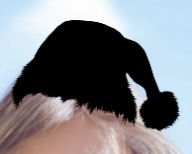# 提取帽子区域
hat = cv2.bitwise_and(resized_hat,resized_hat,mask = mask)#### 五、添加圣诞帽

            # 相加之前保证两者大小一致（可能会由于四舍五入原因不一致）
hat = cv2.resize(hat,(bg_roi.shape,bg_roi.shape))
# 两个ROI区域相加

# 把添加好帽子的区域放回原图
img[y+dh-resized_hat_h:y+dh,(eyes_center-resized_hat_w//3):(eyes_center+resized_hat_w//3*2)] = add_hat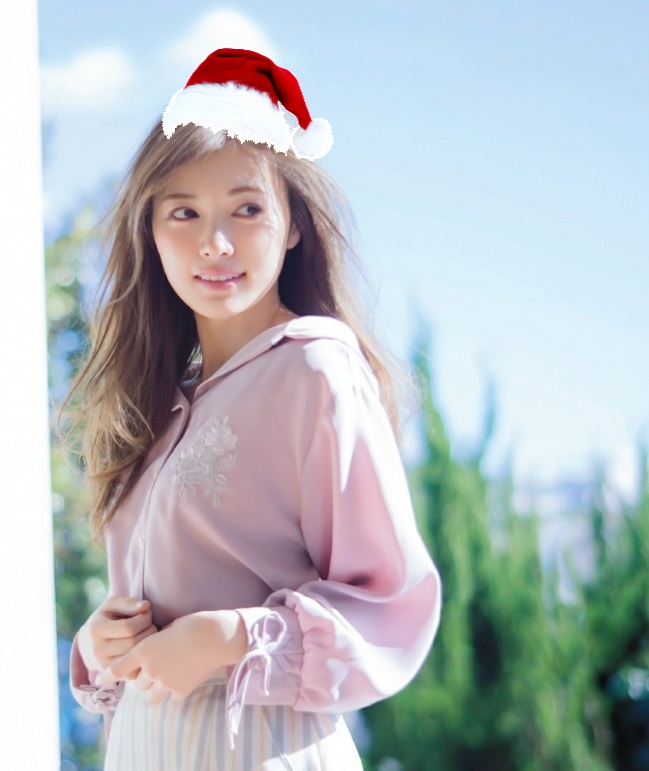12-2686712-245103
12-04225
12-241132
12-25281
09-20
03-021664
12-224
02-23160
12-08670
12-248204
12-251362
12-271万+
12-031